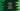# Python program to print a cross pattern using star## How to print a cross pattern in Python:

In this post, we will learn how to print a cross pattern in Python using star or *. This program will take the height as input from the user and it will print the pattern.

You can also use any other character to print this pattern. I will explain you the steps to write the algorithm for this pattern. You can use the same algorithm with any programming language.

### Algorithm to print a cross pattern:

The cross pattern looks as like below:

``````*       *
*     *
*   *
* *
*
* *
*   *
*     *
*       *``````

This is a pattern of height 9. To find the algorithm to print this pattern, let’s replace all blank spaces with #:

``````*#######*
#*#####*#
##*###*##
###*#*###
####*####
###*#*###
##*###*##
#*#####*#
*#######*``````

It is the same pattern, the only difference is that spaces are replaced by #.

Let’s say the row starts from 1 and ends at 9. Similary, column starts at 1 and ends at 9.

We are printing *,

• i = 1 & j = 1 and i = 1 & j = 9
• i = 2 & j = 2 and i = 2 & j = 8
• i = 3 & j = 3 and i = 3 & j = 7 etc.

If you look closely, we are printin the character or * if:

• i and j are equal
• The value of i + j is equal to height + 1.

So, The program we will write will print * if

• i and j are equal
• i + j is equal to height + 1

Other than that, it will print a blank space.

• Use a for loop to run from 1 to height
• Use another inner for loop to run from 1 to height.
• If the variables used for both loops are equal, print *
• If the sum of the variables is equal to height + 1, print *
• Else, print a blank space.
• At the end of the inner for loop, add a newline.

That’s all. Now, let’s write down the program.

### Python program to print a cross pattern:

``````height = int(input('Enter the height of the cross pattern: '))

for i in range(1, height + 1):
for j in range(1, height + 1):
if i == j or i + j == height + 1:
print('*', end='')
else:
print(' ', end='')
print()``````

Here,

• We are taking the height of the cross pattern as input from the user. This value is saved in the height variable.
• The outer for loop is running from i = 1 to i = height.
• The inner for loop is running from j = 1 to j = height.
• If i is equal to j or if the value of i + j is equal to height + 1, it prints *. We are using end=” in the print statement to make sure that it will not add any newline at the end of printing.
• Else, it prints a white space.
• Once the inner for loop ends, it adds a new line.

### Sample output:

This program will print output as like below:

``````Enter the height of the cross pattern: 7
*     *
*   *
* *
*
* *
*   *
*     *

Enter the height of the cross pattern: 8
*      *
*    *
*  *
**
**
*  *
*    *
*      *``````